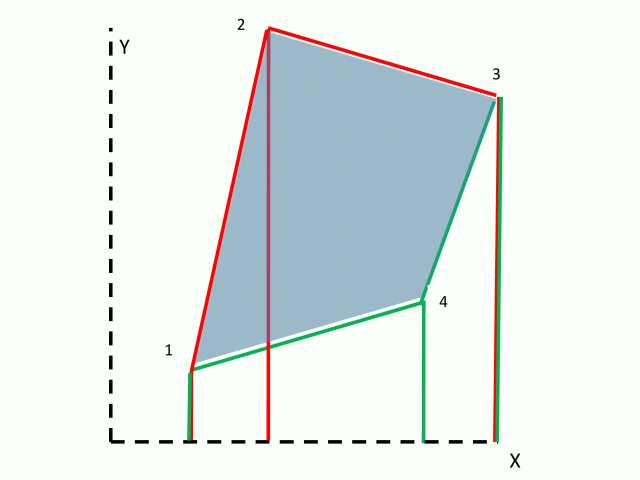## Section Properties from Coordinates

The area of irregular closed polygons defined by coordinates may be conveniently calculated by summing the areas of adjacent trapeziums, as shown below.   The area of each trapezium is calculated from the X axis.  Note that with the apex coordinates defined in a clockwise direction the area between the bottom of the polygon and the x-axis is first added in (red trapeziums), then deducted (green trapeziums), leaving the correct area for the polygon, shaded blue. Other section properties, such as first and second moment of area, may be found in the same way, taking care to ensure that the formula gives a positive value for a positive XD, and negative for negative XD, for line segments above the X axis, and vice versa for segments below the X axis.Voided shapes may be defined by connecting any external apex to one apex of the internal void, then listing the void apex coordinates in an anti-clockwise direction, then finally returning to the external apex.  This procedure is shown for a regular hollow octagon below, but may also be used for any irregular shape, with any number of voids.This entry was posted in Maths, Newton and tagged , , , , . Bookmark the permalink.

### 2 Responses to Section Properties from Coordinates

1.LAction says:

Thanks doug!

This is the same method I used to get the centroid of a polygon as part of an app I’m building since 2011. This process is the same one it’s used to calculate locations by modern GPS systems. My app does exactly the same, but applied to topography.

Here’s part of the code of this method:

Function Centroid(oRng As Range, Optional sType As String) As Variant

Dim vCoords As Variant
Dim vRow() As Variant
Dim i As Long
Dim Area As Double
Dim xPos As Double, yPos As Double

vCoords = oRng.Value

Redim vRow(LBound(vCoords, 2) To UBound(vCoords, 2))

For i = LBound(vCoords, 2) To UBound(vCoords, 2)
vRow(i) = vCoords(1, i)
Next i

Area = CalcArea(vCoords) ‘Note that this is a signed area; if the points are numbered in clockwise order then the area will have a negative sign

xPos = CalcxPos(vCoords, Area)
yPos = CalcyPos(vCoords, Area)

If UCase(sType) = “X” Then
Centroid = xPos
ElseIf UCase(sType) = “Y” Then
Centroid = yPos
ElseIf UCase(sType) = “AREA” Then
Centroid = Abs(Area)
ElseIf UCase(sType) = “SAREA” Then
Centroid = Area
Else
Centroid = “(” & Round(xPos, 3) & “,” & Round(yPos, 3) & “)”
End If

End Function
Private Function CalcxPos(vCoords As Variant, Area As Double) As Double
Dim i As Long

For i = 1 To UBound(vCoords, 1) – 1
CalcxPos = CalcxPos + (vCoords(i, 1) + vCoords(i + 1, 1)) * (vCoords(i, 1) * vCoords(i + 1, 2) – vCoords(i + 1, 1) * vCoords(i, 2))
Next i

CalcxPos = CalcxPos / (6 * Area)

End Function
Private Function CalcyPos(vCoords As Variant, Area As Double) As Double
Dim i As Long

For i = 1 To UBound(vCoords, 1) – 1
CalcyPos = CalcyPos + (vCoords(i, 2) + vCoords(i + 1, 2)) * (vCoords(i, 1) * vCoords(i + 1, 2) – vCoords(i + 1, 1) * vCoords(i, 2))
Next i

CalcyPos = CalcyPos / (6 * Area)

End Function
Private Function CalcArea(vCoords As Variant) As Double
Dim i As Long

For i = 1 To UBound(vCoords, 1) – 1
CalcArea = CalcArea + vCoords(i, 1) * vCoords(i + 1, 2) – vCoords(i + 1, 1) * vCoords(i, 2)
Next i

CalcArea = 0.5 * CalcArea

End Function
Private Function AddRow(InputArr As Variant, vRow As Variant) As Variant
Dim vTemp As Variant
Dim i As Long

If LBound(vRow) LBound(InputArr, 2) Or UBound(vRow) UBound(InputArr, 2) Then AddRow = 0: Exit Function

vTemp = TransposeArray(InputArr)

Redim Preserve vTemp(LBound(vTemp, 1) To UBound(vTemp, 1), LBound(vTemp, 2) To UBound(vTemp, 2) + 1)

vTemp = TransposeArray(vTemp)

For i = LBound(vTemp, 2) To UBound(vTemp, 2)
vTemp(UBound(vTemp, 1), i) = vRow(i)
Next i

End Function
Private Function TransposeArray(InputArr As Variant) As Variant

Dim RowNdx As Long
Dim ColNdx As Long
Dim LB1 As Long
Dim LB2 As Long
Dim UB1 As Long
Dim UB2 As Long
Dim OutputArr() As Variant

LB1 = LBound(InputArr, 1)
LB2 = LBound(InputArr, 2)
UB1 = UBound(InputArr, 1)
UB2 = UBound(InputArr, 2)

Redim OutputArr(LB2 To LB2 + UB2 – LB2, LB1 To LB1 + UB1 – LB1)

For RowNdx = LB2 To UB2
For ColNdx = LB1 To UB1
OutputArr(RowNdx, ColNdx) = InputArr(ColNdx, RowNdx)
Next ColNdx
Next RowNdx

TransposeArray = OutputArr

End Function

Like

This site uses Akismet to reduce spam. Learn how your comment data is processed.